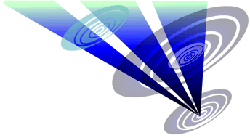Reconstruction of the 2021 Spiers Lane formation 1.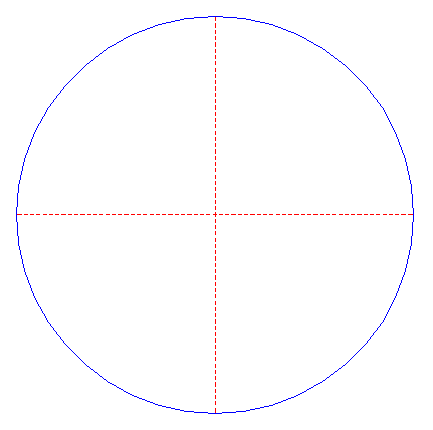Draw a circle. Draw the horizontal and vertical centerlines. 2.Construct the inscribed regular 16-sided polygon of circle 1, pointing up. 3.Draw the sixteen rays of polygon 2, from the center to the angular points. Number these rays (and angular points) 1 - 16, clockwise, starting at the top. 4.Draw the diagonal of polygon 2 between angular points nrs. 1 and 7. 5.Draw the diagonal of polygon 2 between angular points nrs. 16 and 6. 6.Construct a circle concentric to circle 1, passing through the intersection of diagonals 4 and 5. 7.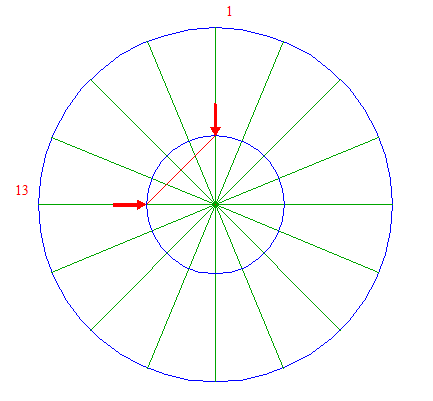Draw the connecting line between the intersections of circle 6 with rays 3 nrs. 1 and 13. 8.Construct a circle concentric to circle 1, tangent to line 7. 9.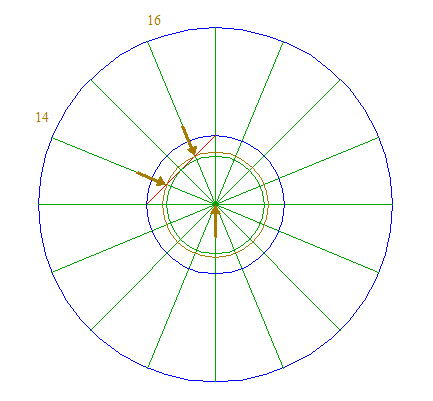Construct a circle concentric to circle 1, passing through the intersections of line 7 with rays 3 nrs. 14 and 16. 10.Construct the circumscribed square of circle 6, with one angular point on ray 3 nr. 3. 11.Construct the circumscribed circle of square 10. 12.Draw two connecting lines, between angular points nrs. 1 and 3, and 2 and 4. 13.Construct a "two-points" circle (defined by the two end-points of a centerline) between the intersections of rays 3 nrs. 2 and 3 with lines 12, as shown. 14.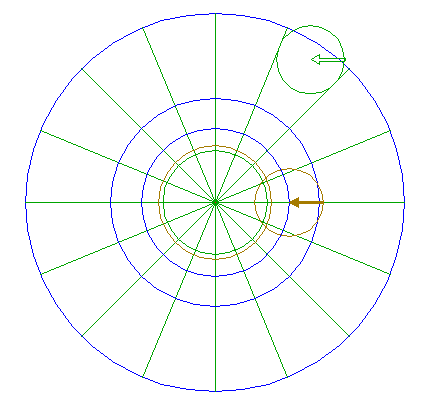Copy circle 13 to the righthand intersection of circle 6 and the horizontal centerline. 15.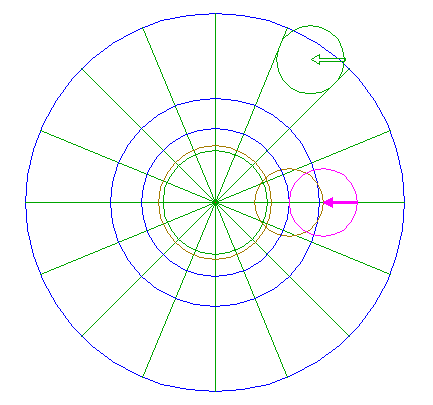Copy circle 13 to the righthand intersection of circle 14 and the horizontal centerline. 16.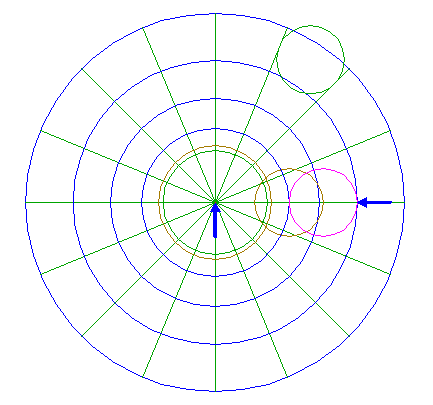Construct a circle concentric to circle 1, tangent to circle 15 at the righthand side. 17.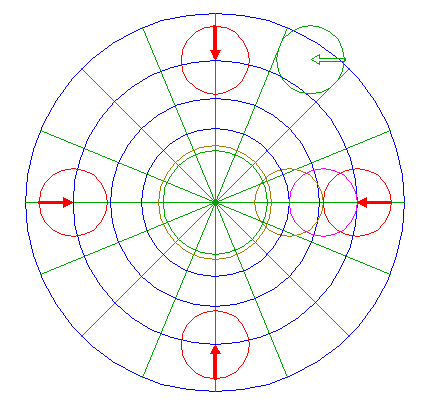Copy circle 13 four times, to the intersections of circle 16 and the horizontal and vertical centerlines. 18.Construct a circle centered at the lower intersection of circles 6 and 14, tangent to circle 15 at the near side. 19.Copy circle 18 to the center of circle 15. 20.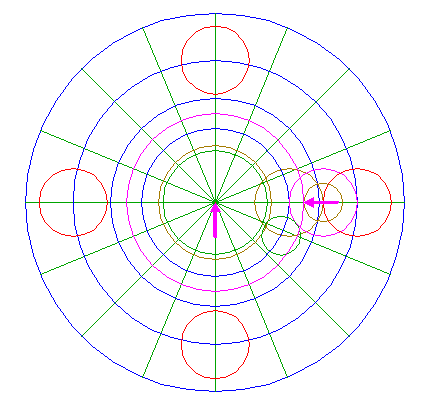Construct a circle concentric to circle 1, tangent to circle 19 at the lefthand side. 21.Copy circle 18 four times, to the intersections of circle 16 with rays 3 nrs. 3, 7, 11, and 15. 22.Draw the connecting line between the intersections of circle 8 with rays 3 nrs. 1 and 10. 23.Construct a circle concentric to circle 1, tangent to line 22. 24.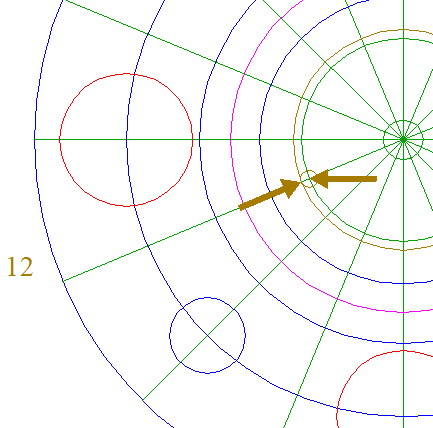Construct a circle centered at the intersection of circle 8 and ray 3 nr. 12, tangent to circle 9 at the near side. 25.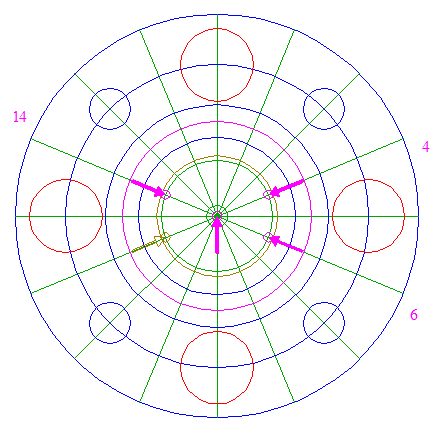Copy circle 24 four times, to the center of circle 1, and to the intersections of circle 8 with rays 3 nrs. 4, 6, and 14. 26.Draw four lines, two parallel to rays 3 nrs. 4 and 14, tangent to circles 25 at the lower sides, and two parallel to rays 3 nrs. 6 and 12, tangent to circles 24 and 25 at the upper sides. See detail.27.Construct a circle centered at the lower intersection of circle 23 and the vertical centerline, tangent to the central circle 25 at the lower side. 28.Copy circle 27 to the righthand intersection of circle 1 and the horizontal centerline. 29.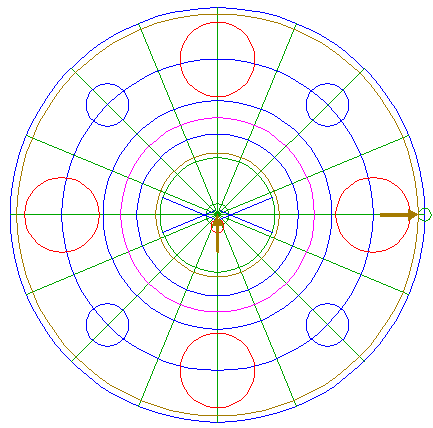Construct a circle concentric to circle 1, tangent to circle 28 at the lefthand side. 30.Construct a "two-points" circle between the lower intersections of circles 23 and 27 with the vertical centerline. 31.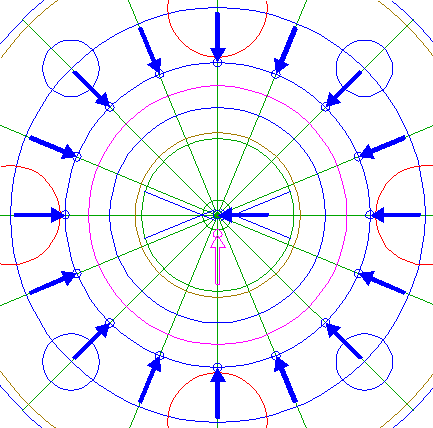Copy circle 30 seventeen times, to the center of circle 1, and to the intersections of circle 11 and rays 3. 32.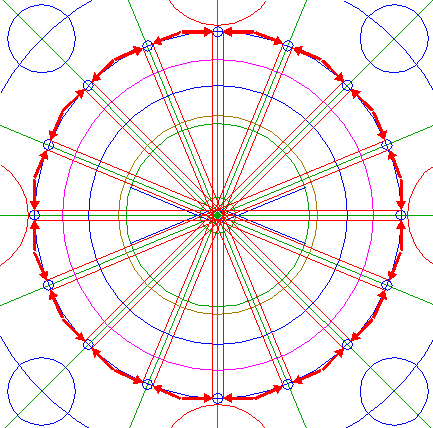Draw sixteen pairs of parallel lines, parallel to rays 3, tangent at both sides to the central circle 31 and the other circles 31, as shown. 33.Draw the connecting line between the righthand intersections of circles 6 and 20 with the horizontal centerline, and divide this line into five equal parts. 34.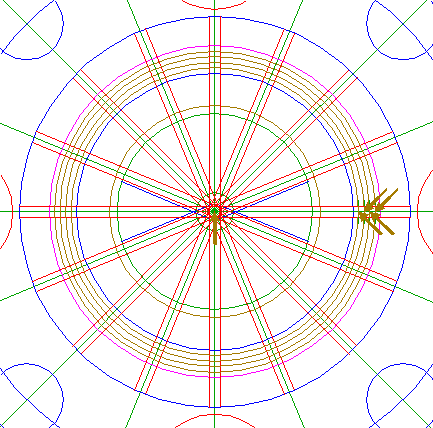Construct four circles concentric to circle 1, passing through dividing nodes 33. See detail.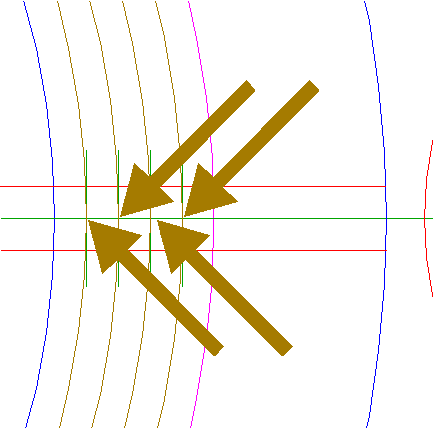35.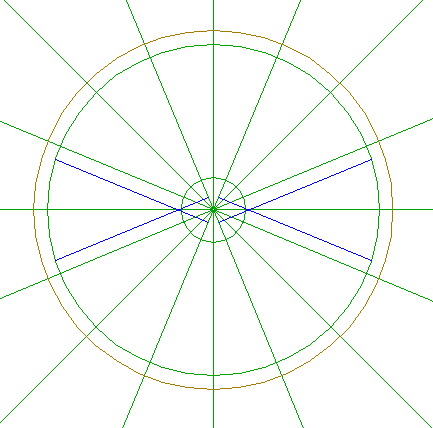Remove all redundant parts from lines 3, and 26, and from circles 8, 9, and 23, such that the shown patterns remain.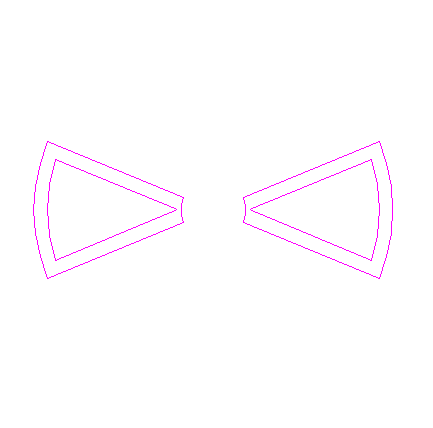36.Remove all redundant parts from circles 6, 20, and 34, and from lines 32, such that the shown patterns remain.37.Circles 1, 11, 16, 17, 21, and 29, and patterns 35, and 36, are used for the final reconstruction. 38.Remove all parts not visible within the formation itself. 39.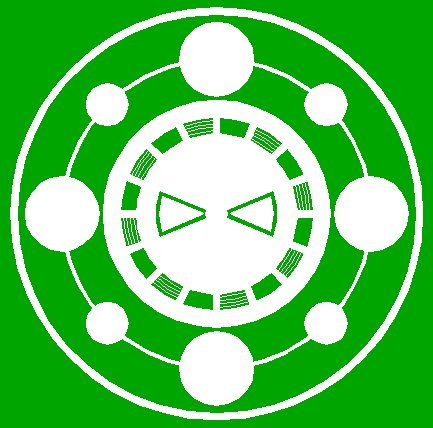Colour all areas corresponding to standing... 40....or to flattened crop, and finish the reconstruction of the 2021 Spiers Lane formation. 41.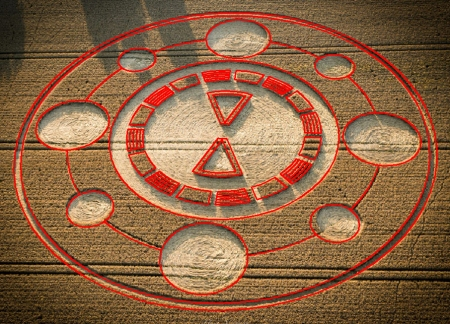The final result, matched with two aerial images.